An imbalanced dataset is a dataset where there’s a substantial mismatch between the number of records belonging to each category. Real-world datasets can be highly imbalanced, which may affect performance of statistical algorithms or machine learning models.

In this tutorial, we’ll study downsampling and upsampling, which are the two main techniques for handling imbalanced datasets. We’ll show you how to downsample with the sklearn library and how to upsample with sklearn and the SMOTE library for Python.

The CSV file containing the sample dataset for this article can be downloaded from this kaggle link. It’s been mirrored on this site if you’d like to download it directly. The dataset consists of ham and spam text messages.

``````import pandas as pd
import matplotlib.pyplot as plt
import numpy as np
import seaborn as sns

sns.set_style("darkgrid")
sns.set_context("poster")
plt.rcParams["figure.figsize"] = [8,6]``````

If you don’t already have these libraries installed, you can install them with the following PIP commands:

``````pip install numpy
pip install matplotlib
pip install scikit-learn
pip install seaborn``````

The script below imports the CSV file containing the dataset using the `read_csv()` method from the Pandas module.

The dataset consists of 5 columns by default but we filter the dataset since we’re only interested in columns v1 and v2. Column v1 contains labels v2 has the corresponding text message. The script also prints the dataset header via the `head()` method of the Pandas dataframe so we can preview our dataset.

``````spam_dataset = pd.read_csv(r"C:\Datasets\spam.csv", encoding = 'latin')
spam_dataset = spam_dataset[["v1", "v2"]]

Output: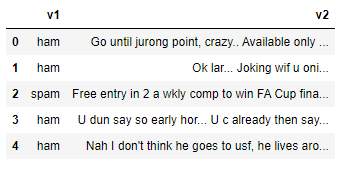The v1 column contains labels or categories for the messages in our dataset. Let’s see how the data is distributed for the different categories. The script below prints the count of messages for each category along with a pie chart depicting the distribution.

``````print(spam_dataset["v1"].value_counts())

spam_dataset.groupby('v1').size().plot(kind='pie',
y = "v1",
label = "Type",
autopct='%1.1f%%')``````

Output: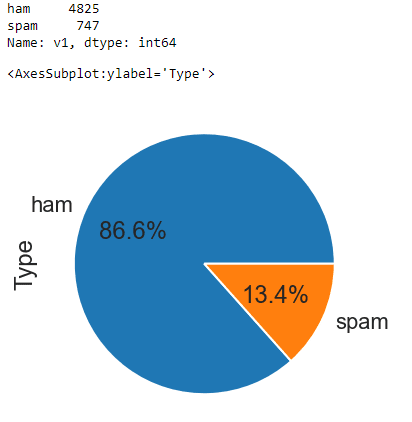From the above output, you can see that there are 4825 ham messages in our dataset, while the number of spam messages is only 747. The pie chart further highlights the imbalanced nature of our dataset where 86.6% of our records belong to the ham category while only 13.4% of our records are spam.

Before we show you how to balance this dataset, let’s divide our dataset into two parts: one containing ham messages and the other containing spam messages. Run the script below to do so:

``````ham_messages = spam_dataset[spam_dataset["v1"] == "ham"]
spam_messages  = spam_dataset[spam_dataset["v1"] == "spam"]
print(ham_messages.shape)
print(spam_messages.shape)``````

Output:

``````(4825, 2)
(747, 2)``````

I put together a Python Developer Kit with over 100 pre-built Python scripts covering data structures, Pandas, NumPy, Seaborn, machine learning, file processing, web scraping and a whole lot more - and I want you to have it for free. Enter your email address below and I'll send a copy your way.

Yes, I'll take a free Python Developer Kit

## Downsampling with sklearn

Downsampling refers to removing records from majority classes in order to create a more balanced dataset. The simplest way of downsampling majority classes is by randomly removing records from that category. Let’s walk through an example.

The script below calls the `resample()` method from the sklearn.utils module for downsampling the ham class. The dataset containing the ham messages is passed as the first argument to the method. The `replace = True` attribute performs random resampling with replacement. The `n_samples` attribute defines the number of records you want to select from the original records. We have set the value of this attribute to the number of records in the spam dataset so the two sets will be balanced.

``````from sklearn.utils import resample
ham_downsample = resample(ham_messages,
replace=True,
n_samples=len(spam_messages),
random_state=42)

print(ham_downsample.shape)``````

Output:

``(747, 2)``

Next, to create a final dataset, you can concatenate your original spam dataset with the down-sampled ham dataset. The following script concatenates the two datasets and again prints the class distribution and a pie chart for the ham and spam messages.

``````data_downsampled = pd.concat([ham_downsample, spam_messages])

print(data_downsampled["v1"].value_counts())

data_downsampled.groupby('v1').size().plot(kind='pie',
y = "v1",
label = "Type",
autopct='%1.1f%%')``````

Output: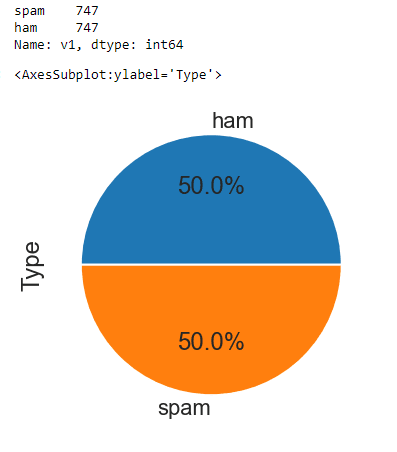From the above output, you can see that the number of records in both ham and spam categories is 747 - equal to the original number of spam messages. The pie chart confirms the data is now evenly distributed between our two message categories.

## Upsampling with sklearn

Upsampling refers to manually adding data samples to the minority classes in order to create a more balanced dataset.

In this section, you’ll see two techniques for upsampling.

### Upsampling By Copying Minority Class Instances

You can upsample a dataset by simply copying records from minority classes. You can do so via the `resample()` method from the sklearn.utils module, as shown in the following script.

``````from sklearn.utils import resample
spam_upsample = resample(spam_messages,
replace=True,
n_samples=len(ham_messages),
random_state=42)

print(spam_upsample.shape)``````

You can see that in this case, the first argument we pass the `resample()` method is our minority class, i.e. our spam dataset. The value for the `n_samples` parameter is set to the number of records in the majority class (ham messages) since we want equal representation for both classes in our dataset.

Output:

``(4825, 2)``

From the output above, you can see that the number of spam messages has increased to 4825 - equal to the number of ham messages.

To create our final dataset after upsampling, you can concatenate the original ham messags dataset with the upsampled spam message dataset, as demonstrated in the following script. The script below also shows the class distribution via a pie chart.

``````data_upsampled = pd.concat([ham_messages, spam_upsample])

print(data_upsampled["v1"].value_counts())

data_upsampled.groupby('v1').size().plot(kind='pie',
y = "v1",
label = "Type",
autopct='%1.1f%%')``````

Output: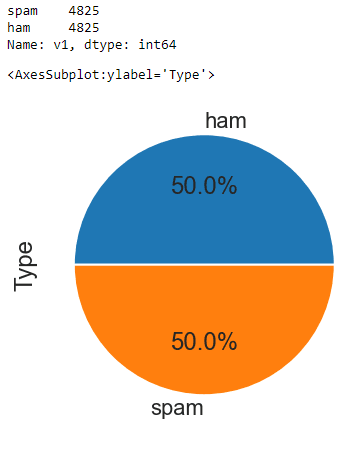I put together a Python Developer Kit with over 100 pre-built Python scripts covering data structures, Pandas, NumPy, Seaborn, machine learning, file processing, web scraping and a whole lot more - and I want you to have it for free. Enter your email address below and I'll send a copy your way.

Yes, I'll take a free Python Developer Kit

### Upsampling with SMOTE

Upsampling by simply copying records may lead to overfitting when you train machine learning models. Techniques have been developed that add instances to dataset which are not exactly the copy of existing instances but are very similar to the original instances.

One such technique is SMOTE - Synthetic Minority Over-sampling Technique. If you’re curious about the math behind this technique, you can read the research paper that proposed SMOTE.

In Python, you can use the imblanced-learn library to apply SMOTE upsampling. Install the imblanced-learn library with the following PIP command:

``pip install imbalanced-learn``

SMOTE, like other statistical algorithms, works with numerical data and requires both feature and label sets. In our dataset, the feature set consists of text messages. You need to convert this text to numeric form before you can apply SMOTE. One way to convert text to numbers is with TFIDF vectorization, which is available in sklearn.

You can call the `TfidfVectorizer` class from the sklearn.feature_extraction.text submodule to convert text to something numeric. You have to pass the dataframe column containing your text to the `fit_transform()` method of the `TfidfVectorizer` object, as shown in the script below.

``````from sklearn.feature_extraction.text import TfidfVectorizer
tfidf = TfidfVectorizer()
X = tfidf.fit_transform(spam_dataset ['v2'])``````

Our label set also consists of text labels, ham and spam. You need to convert these to numbers as well. This next script replaces the label ham with 0, and the label spam with 1 in the v1 column of our dataset.

``````spam_dataset['v1'] = spam_dataset['v1'].map({'ham': 0, 'spam': 1})

Output: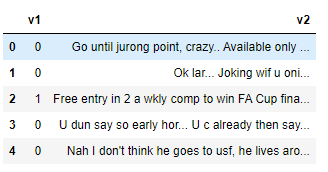You can now see from the above dataset header that the v1 column consists of digits.

Finally, you can create a label set by filtering values from the v1 column. Here’s the script for that:

``y = spam_dataset[['v1']]``

We have converted both feature set (X), and label set (y) to numeric form so now we’re ready to apply SMOTE for upsampling our dataset.

The following script imports the `SMOTE` class from the imblearn.over_sampling module. To perform SMOTE, you need to pass your feature and label sets to the `fit_resample` sample method of the `SMOTE` class object.

``````from imblearn.over_sampling import SMOTE

su = SMOTE(random_state=42)
X_su, y_su = su.fit_resample(X, y)``````

Finally, you can print your new class distribution using the following script:

``````print(y_su["v1"].value_counts())

y_su.groupby('v1').size().plot(kind='pie',
y = "v1",
label = "Type",
autopct='%1.1f%%')``````

Output: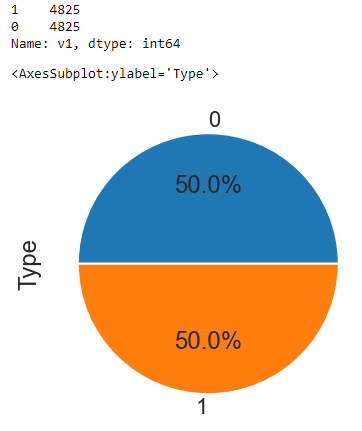The above output shows that the SMOTE algorithm has successfully applied over-sampling to the minority class (spam messages) in our dataset, which has resulted in a balanced dataset.

#### Python SMOTE Upsampling - Complete Code

Since it’s a bit more complicated than upsampling with sklearn, I’ve included the complete code for SMOTE upsampling below:

``````import pandas as pd
import matplotlib.pyplot as plt
import numpy as np
import seaborn as sns
from imblearn.over_sampling import SMOTE
from sklearn.feature_extraction.text import TfidfVectorizer

sns.set_style("darkgrid")
sns.set_context("poster")
plt.rcParams["figure.figsize"] = [8,6]

#import file
spam_dataset = pd.read_csv(r"C:\Datasets\spam.csv", encoding = 'latin')
spam_dataset = spam_dataset[["v1", "v2"]]

print("Before Upsampling:")
print(spam_dataset["v1"].value_counts())

#convert text to numbers
tfidf = TfidfVectorizer()
X = tfidf.fit_transform(spam_dataset ['v2'])

#convert labels to numbers
spam_dataset['v1'] = spam_dataset['v1'].map({'ham': 0, 'spam': 1})

#extract label set
y = spam_dataset[['v1']]

#Use SMOTE for upsampling
su = SMOTE(random_state=42)
X_su, y_su = su.fit_resample(X, y)

print("After Upsampling:")
print(y_su["v1"].value_counts())

y_su.groupby('v1').size().plot(kind='pie',
y = "v1",
label = "Type",
autopct='%1.1f%%')``````

If you liked this tutorial and want more tips for getting the most out of Python, please subscribe using the form below.